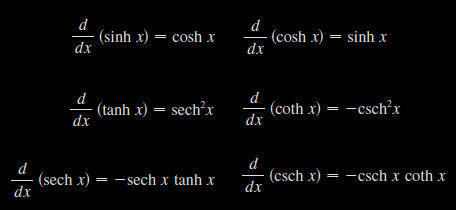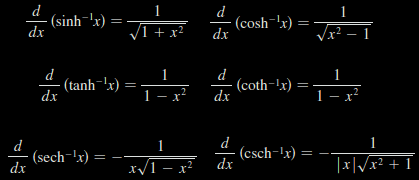# Stewart – Calculus – 5.3 – The Fundamental Theorem of Calculus

The Fundamental Theorem of Calculus, Part 1

The Fundamental Theorem of Calculus, Part 2

# Stewart – Calculus – 5.1 / 5.2 – Areas and Distances / The Definite Integral

Approximating Area Using Rectangles

The Definite Integral

Calculating a Definite Integral Using Riemann Sums — Part 1

Calculating a Definite Integral Using Riemann Sums — Part 2

Three equations that give formulas for sums of powers of positive integers: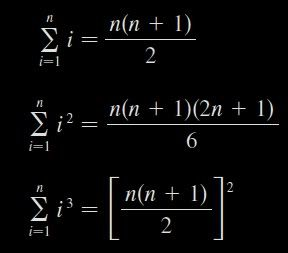These formulas are simple rules for working with sigma notation: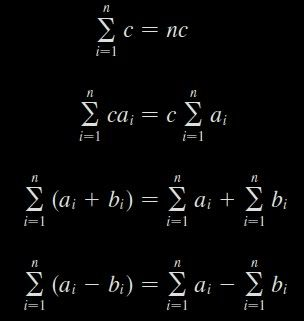Use the form of the definition of the integral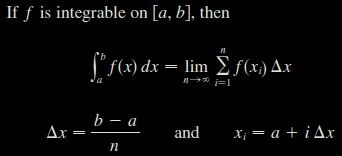to evaluate the integral.$\int_{1}^{2} x^3 dx$# Stewart – Calculus – 4.5 – Summary of Curve Sketching

Mnemonic Device for Remembering Steps in Curve Sketching

A. Domain
B. Intercepts
C. Symmetry
D. Asymptotes
E. Decrease or Increase
F. Local Maximum and Minimum
G. Concavity and Points of InflectionDill Chips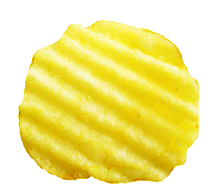“This is a dill chip.”

Now, think of this phrase as, “Dis a dill chip.”
This phrase is close to, “DISADILCIP.”

Use the first letters to reconstruct the steps: Domain, Intercepts, Symmetry, Asymptotes, Decrease/Increase (intervals), Local max/min, Concavity, Inflection Point.

(If you think of something better, please, share it.)

# Stewart – Calculus – 3.11 – Hyperbolic Functions

Quizlet Quiz